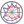Course title
Linear Algebra 1
 YAMAZAWA HiroshiHORIUCHI Tomohiro HORIBE Tadashi
Course description
Linear algebra is an algebraic and geometric theory of properties called linearity. This method is the basis of various fields such as engineering and statistics, and the problem in these fields is often reduced to a problem of linear algebra.
In this class, students learn about matrices and determinants with the goal of solving system of linear equations, and also learn about diagonalization.
Purpose of class
The purpose of this class is to understand the concept of matrices and determinants, and to be able to calculate them.
Goals and objectives
1. To be able to calculate operations for matrices.
2. To be able to solve system of linear equations.
3. To be able to calculate determinants.
4. To be able to calculate diagonalization.
Language
Japanese
Class schedule

Class schedule HW assignments (Including preparation and review of the class.) Amount of Time Required
1. Matrix and its operations Read through the textbook p.13-p.21 and handouts, and solve the exercises before class. Review after class. 190minutes
2. Square matrix of order 2 and its applications Read through the textbook p.5-p.11 and handouts, and solve the exercises before class. Review after class. 190minutes
3. Inverse and transpose matrix Read through the textbook p.21-p.27 and handouts, and solve the exercises before class. Review after class. 190minutes
4. Elementary row operations Read through the textbook p.31-p.37 and handouts, and solve the exercises before class. Review after class. 190minutes
5. Row echelon form and solving system of linear equations Read through the textbook p.37-p.46 and handouts, and solve the exercises before class. Review after class. 190minutes
6. Calculation of inverse matrix Read through the textbook p.46-p.48 and handouts, and solve the exercises before class. Review after class. 190minutes
7. Midterm examination and its explanation Preparation of midterm examination. 190minutes
8. Determinant of matrix of order 2 and 3, and permutation Read through the textbook p.51-p.60 and handouts, and solve the exercises before class. Review after class. 190minutes
9. Definition and basic properties of determinant Read through the textbook p.60-p.74 and handouts, and solve the exercises before class. Review after class. 190minutes
10. Cramer's rule and cofactor Read through the textbook p.74-p.80 and handouts, and solve the exercises before class. Review after class. 190minutes
11. Calculation of determinant Read through the textbook p.60-p.80 and handouts, and solve the exercises before class. Review after class. 190minutes
12. Eigenvalue and eigenvector Read through the textbook p.157-p.164 and handouts, and solve the exercises before class. Review after class. 190minutes
13. Diagonalization of square matrix Read through the textbook p.164-p.168 and handouts, and solve the exercises before class. Review after class. 190minutes
14. Final examination and its explanation Preparation of final examination. 190minutes
Total. - - 2660minutes
Relationship between 'Goals and Objectives' and 'Course Outcomes'

Midterm examination Final examination Reports and little examinations Total.
1. 17% 25% 42%
2. 3% 5% 8%
3. 13% 20% 33%
4. 7% 10% 17%
Total. 20% 20% 60% -
Evaluation method and criteria
Midterm examination(20%), Final examination(20%), Reports and little examinations(60%)
60% if students can understand and solve exercises in the handouts
Textbooks and reference materials
Textbook: 工学系のための線形代数、黒川康宏、佐々木真二、廣瀬三平、山澤浩司、東京図書
Prerequisites
Review high school mathematical contents
Office hours and How to contact professors for questions
• 30 minutes after class
Regionally-oriented
Non-regionally-oriented course
Development of social and professional independence
• Course that cultivates an ability for utilizing knowledge
Active-learning course
Most classes are interactive
Course by professor with work experience
Work experience Work experience and relevance to the course content if applicable
N/A 該当しない
Education related SDGs:the Sustainable Development Goals• 9.INDUSTRY, INNOVATION AND INFRASTRUCTURE